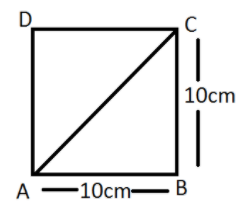QuestionAnswers

# Find the diagonal of a square whose side is $10cm$.Verified
128.7k+ views
Hint:As we know the side of the given square is $10cm$. And in a square, all the four sides are equal to each other as well as all the sides interest to its adjacent side at ${90^0}$. Therefore, the figure formed by joining the two opposite vertices of a square, is a right-angled triangle with two equal sides.

Complete step-by- step solution:Given that Side of the square is $10cm$
In right angled$\vartriangle ABC$
Using Pythagoras theorem
$A{B^2} + B{C^2} = A{C^2}$ $\left[ {Bas{e^2} + Perpendicular{r^2} = Hypotenuse{e^2}} \right]$
As we know, the sides of a square are equal to each other.
$\Rightarrow$ $AB = BC$
$\Rightarrow$${10^2} + {10^2} = A{C^2}$
$\Rightarrow$$100 + 100 = A{C^2}$
$\Rightarrow$$200 = A{C^2}$
$\Rightarrow$$AC = \sqrt {200}$
$\Rightarrow$$AC = \sqrt {2 \times 2 \times 2 \times 5 \times 5}$
$\Rightarrow$$AC = 2 \times 5\sqrt 2$
$\Rightarrow$$AC = 10\sqrt 2$
Therefore, the diagonal of square will be $10\sqrt 2$

Note: A square can have two diagonals. Each of the diagonal can be formed by joining the diagonally opposite vertices of a square. The properties of diagonals are as follows-
Both the diagonals are congruent (same length). Both the diagonals bisect each other, i.e. the point of joining of the two diagonals is the midpoint of both the diagonals. A diagonal divides a square into two isosceles right-angled triangles. The sum of all the internal angles of a square is equal to $360 \circ$and a square is a regular quadrilateral that has four equal sides and four same angles.
The diagonal of a square with side ‘a’ can be calculated using a formula $a\sqrt 2$. Remember, both the diagonals of a square are equal to each other.